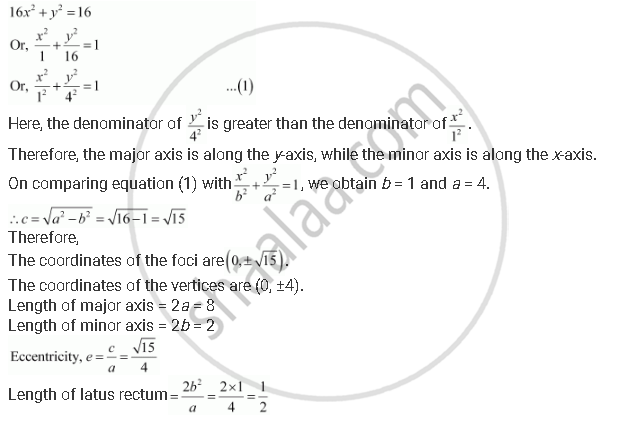Share

# Find the Coordinates of the Foci, the Vertices, the Length of Major Axis, the Minor Axis, the Eccentricity and the Length of the Latus Rectum of the Ellipse 16x2 + Y2 = 16 - Mathematics

#### Question

Find the coordinates of the foci, the vertices, the length of major axis, the minor axis, the eccentricity and the length of the latus rectum of the ellipse 16x2 + y2 = 16

#### Solution

The given equation is 16x2 + y2 = 16.

It can be written asIs there an error in this question or solution?